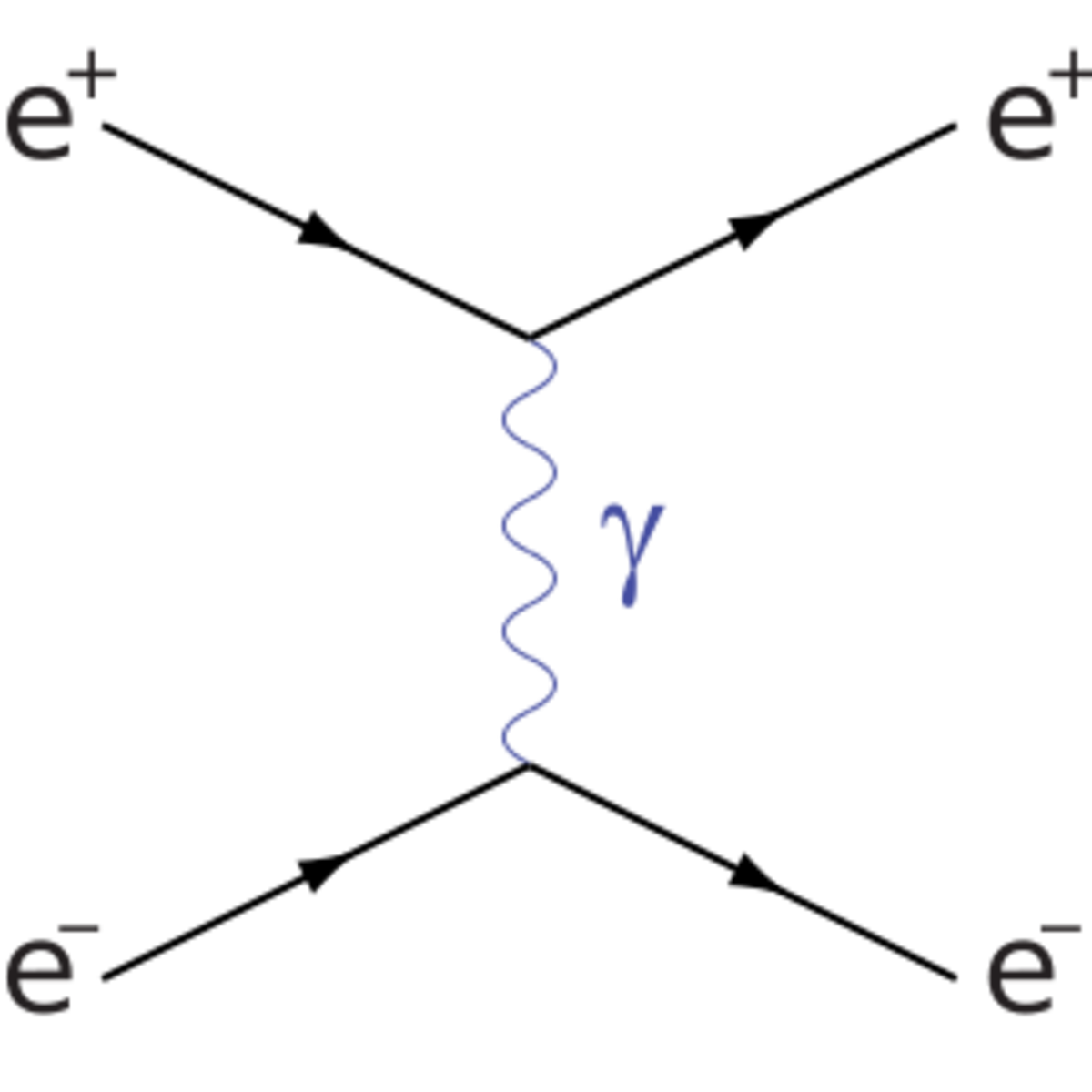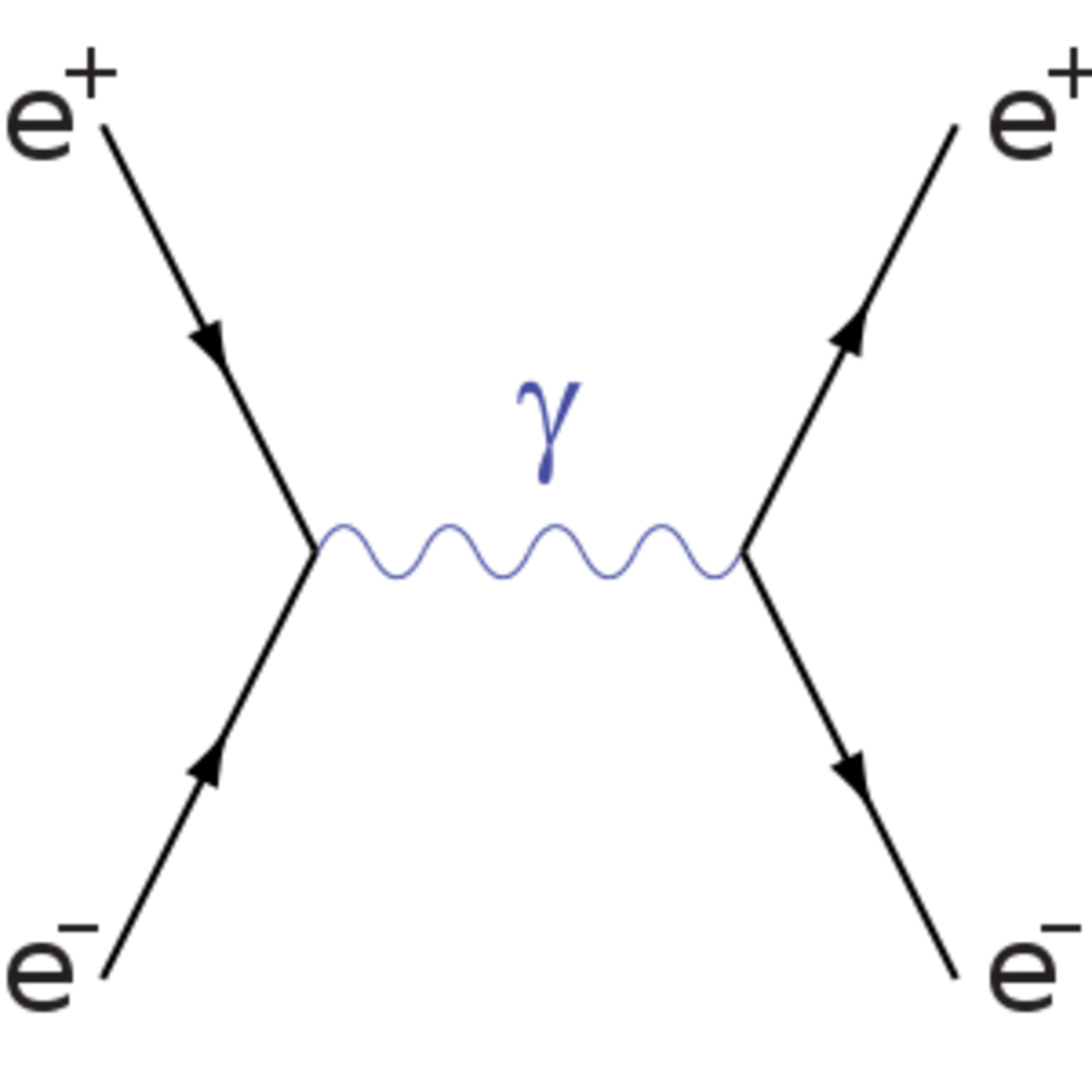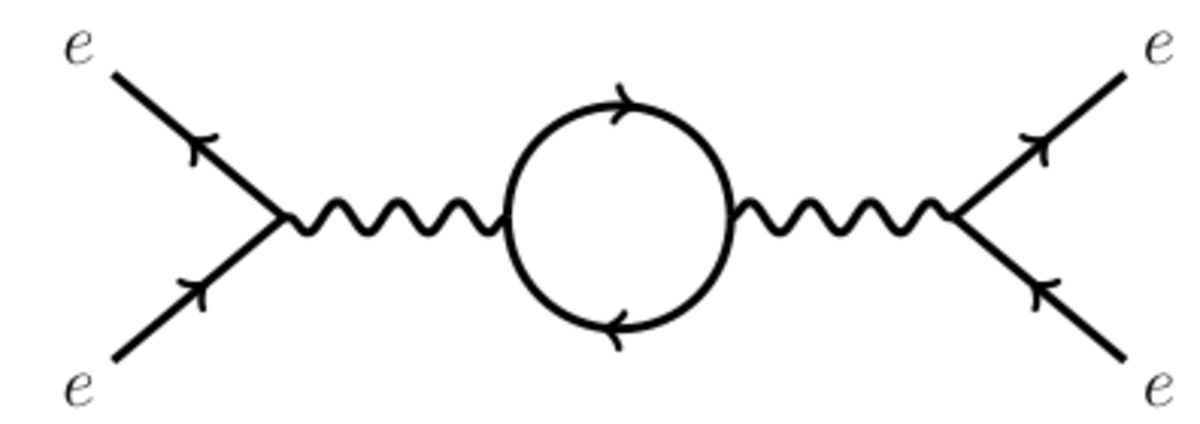Updated date:

# Feynman Diagrams: An Introduction

Author:

Feynman diagrams are used in quantum field theory and particle physics to represent interactions between elementary particles and calculate the probability of these interactions occurring.

In quantum particle physics, each particle is represented by a quantum field. To calculate how the particles interact we need to perform a complex calculation in quantum field theory. A Feynman diagram is a way of doing the calculation pictorially, saving a lot of time and effort.

As an example, imagine that we want to calculate the amplitude of the electron-positron scattering reaction: e- + e+ → e- + e+ (Bhabha scattering).

## Key to Feynman Diagrams

Time runs from left to right.

A straight line represents a fermion (an electron or positron).

A wavy line represents a photon.Diagram 1: electron and positron scatter by exhcanging a photonDiagram 2: electron-positron annihilation

## Example: Bhabha Scattering

We know that we start with an electron and a positron. We also know that at the end of the process we again have an electron and a positron. What we can't know for certain is what happens in between. We may have a process where the electron and positron scatter off each other by exchanging a photon, as in Diagram 1. Or, the electron and positron may annihilate to a photon which soon decays back into an electron and a positron, as in Diagram 2.

There are even more complicated possibilities, in which the electron or the positron emits and reabsorbs a photon, shown in Diagram 3. There are infinitely many possibilities because we can keep adding more loops to the diagram.Diagram 3: One-loop diagram for electron-positron annihilation

In quantum mechanics, the probability of a process occurring is given by the amplitude squared, where the amplitude is the sum of all the ways that the process could occur.

In our example, to calculate the probability of the reaction we need to “add up” all the diagrams. First calculate the value of the diagram using the Feynman rules. The Feynman rules tell us the value associated with each straight line (fermion), wavy line (boson) and vertex. To calculate the value of the diagram we simply multiply together the value of each of these consituent parts of the diagram.

This is the beauty of Feynmann diagrams: once you know what each part is worth, it is very easy to work out the probability of the reaction through simple multiplication and addition. The alternative, without the diagrams, would be to perform a lengthy and complicated quantum field theory calculation.

But hang on a minute: we said that we needed to add up an infinite number of diagrams. How can we do that? Well, the simple answer is, we can't. Fortunately, every loop contributes a factor of much less than one to the value of the diagram. This means that diagrams with many loops have a very small value so don't contribute much to the total amplitude of the reaction. We can get a good approximation to the amplitude of e- + e+ → e- + e+ just by adding up adding diagrams 1 and 2. If we need a more accurate result, we can add in the 1-loop diagrams such as diagram 3.

Feynman diagrams are routinely used by particle physicists in this way to calculate scattering amplitudes. It is therefore essential that physics students are confident in the use of Feynman diagrams.

## The Best Physics Books for learning about Feynman Diagrams

Peskin and Schroeder's Introduction to Quantum Field Theory is ultimate textbook for students of theoretical particle physics. I found this invaluable during my degree.

Another excellent quantum field theory textbook by Mark Srednicki.

## More particle physics articles from topquark

topquark works as a researcher in theoretical particle physics and blogs about research at The Particle Pen.

## Comments

againsttheodds on April 30, 2012:

Great explanation of feyman diagrams. Voted up.

dipless from Manchester on December 27, 2011:

A nicely written hub, Richard Feynman was an absolute legend and his work on QED theory and quantum mechanics was amazing. Nice to see a hub written about him.

topquark (author) from UK on July 07, 2011:

Thanks. Maybe I'll do that in a future hub.

Xavier Nathan from Isle of Man on July 07, 2011:

Another clearly explained hub that I found most interesting. I would have liked to have seen an example of how scattering amplitudes are calculated to show how the the diagrams are used in practice. Thank you.